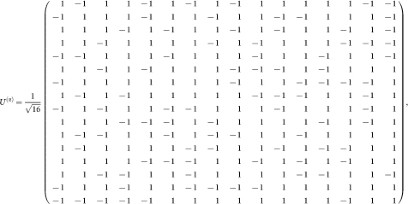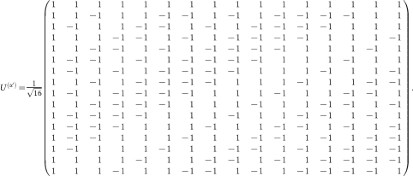## Introduction

Theft of electronic information is a major problem in modern telecommunications. For instance, recent advances in computing technology such as the ever growing parallel processing power of graphical processing units (GPUs), have allowed the brute-force cracking of passwords to be greatly sped up1,2. A very promising solution to the issue of information security is quantum key distribution (QKD), also called quantum cryptography3. Quantum cryptographic schemes allow remote parties to share a secret key (a sequence of bits) through the use of quantum channels. This key is later used for secure information transmission and the advantage of QKD is that the presence of an eavesdropper may, in principle, be detected. The first QKD protocol was proposed in 1984 by Bennett and Brassard, called BB844. It employs two sets of orthogonal quantum states spanning two mutually unbiased bases (MUBs)5. BB84 is a prepare-and-measure QKD protocol, in which single quantum systems are exchanged between the communicating parties (Alice and Bob) for the establishment of each shared secret key bit.

Most experimental implementations of quantum cryptography have been done with BB84-based QKD schemes. Experiments with increased performance (i.e. higher transmission distance, larger secure key generation rate, more compact schemes, etc...) have been demonstrated in the past few years6,7,8,9,10,11 and the vast majority of these demonstrations have employed only two-dimensional quantum systems. The use of high dimensional systems has been nevertheless widely deployed in classical digital communications for many years, as a way to maximize the efficiency of the transmission channel12. With the same goal, the first studies on the theoretical framework to generalize the BB84 protocol to high dimensional state spaces were carried out over a decade ago13,14,15. It was shown that there is an important additional feature in moving to higher dimensions in QKD: increased security, which is alone a major motivation to implement high-dimensional quantum cryptography. Nonetheless, due to experimental challenges, there have been only proof-of-principle experiments of QKD in higher dimensions16,17,18.

Recently, on the theoretical front, the interest on high-dimensional QKD did not wane as security bounds were derived considering finite key lengths19. Moreover, an important experimental tool has emerged in recent years to dynamically manipulate light in free-space, the spatial light modulator (SLM)20. The capabilities of the SLMs have been explored in experiments of quantum information and it is now widely recognized as a tool for the manipulation of high-dimensional quantum systems (qudits) encoded in the transverse momentum of single photons21,22,23,24,25,26. In classical optical communications, SLMs have also been used to multiplex and demultiplex classical high-speed data streams, further increasing the channel capacity27. Nevertheless, in spite of all recent progress, a demonstration of a quantum key exchange session using the BB84 protocol in higher dimensions is still lacking. In this paper this issue is finally addressed.

We report an automated QKD session between Alice and Bob, based on the BB84 protocol extended to 16-dimensional quantum systems15. We employ the linear transverse momentum of single-photons as the degree of freedom for encoding the qudit states28. The single-photons are produced by Alice from attenuated laser pulses. At Alice and Bob's sites, the quantum states spanning the MUBs are randomly produced with the help of SLMs, dynamically introducing relative phases between the paths available for the propagation of the single photons25. The entire setup is synchronized working at a repetition rate of 30 Hz, which is the current physical limit of standard commercially available SLMs. The custom electronics required for the full automated execution of the protocol were developed on field programmable gate array (FPGA) units. The measured quantum bit error rate (QBER) of the correlated bits generated was smaller than half of the bound allowed for extracting a secret key while considering the general coherent attacks; and close to three times smaller than the limit for individual attacks15,19.

## Results

In this demonstration we take advantage of the flexibility allowed by SLMs to encode information using the transverse wave profile of single photons. Although it is a continuous degree of freedom, it can be discretized using slits to define different possible paths for the photon transmission28. The SLMs are built with liquid crystal displays (LCDs) and require the use of polarizers. They allow an individual control of the gray level of each pixel on the LCD29. While changing the pixel gray level, the polarization is changed and when combined with the polarizers, the light transmissivity of the pixel is varied. Together with this effect a relative phase change may also be induced in each pixel. The slits can be directly generated on the SLM. This is done by programming the gray levels of all the LCD pixels such that areas with high transmissivity correspond to the slits and everywhere else has zero transmission. By employing a set of D parallel slits a Hilbert space of D dimensions is constructed. The state after the slits can be written as the following coherent superposition: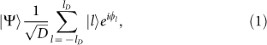where lD = (D − 1)/2. |l〉 represents the state of the photon transmitted by the lth-slit of the SLM28. The uniform amplitude distribution is obtained with an adequate choice of the spatial beam profile on the plane of the slits25. This state can be generated provided that the single-photons have a larger transverse coherence length than the distance between the outermost slits. ϕl are the relative phase shifts at each slit. Each ϕl can be dynamically changed in real-time in the SLMs.

In standard BB84 quantum cryptography, the required states are two vectors of two-dimensional MUBs, with a total of four states required. In the case of a multi-dimensional BB84 protocol, the individual states to be sent and measured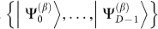belong to two D-dimensional MUBs. The index β denotes a specific MUB with β = α, α′. In this experiment 16 slits are employed, generating a 16-dimensional Hilbert space. Consequently 32 states had to be generated, 16 for each MUB. Of the large number of families of MUBs that exist in 16 dimensions30, we chose two MUBs that only required phase modulations of 0 and π and no amplitude modulation (see Methods). This eased the implementation of the MUBs states with the SLMs.

Alice needs to randomly choose in which MUB, α or α′, she will encode her qudit. She then randomly chooses one of the D possible states from this MUB. As we will explain next, in our scheme, this is done by using a phase-modulation SLM and pseudo-random subroutines to depict a sequence of pre-defined masks. Upon successfully receiving the photon, Bob needs to make a projective measurement. He also randomly chooses states from α and α′. For this purpose, Bob uses a detection apparatus composed of a phase-modulation SLM, a lens and a “point-like” avalanche single photon detector (APD) [shown in Fig. 1(a)]. The single-photon detector remains fixed at the center of the focal plane. It covers the transverse blue zone of 10 μm indicated in Fig. 1. At the SLM, Bob depicts phase-masks where the phases at each slit are chosen in accordance with the MUBs states given in the Methods section. In this case, the probability of single-photon detection is proportional to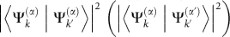when Alice and Bob choose the states belonging to the same (different) MUBs, where k, k′ = 0, …, D − 1. Each time Bob matches his state choice with Alice's, while both choose the same MUB, constructive spatial interference occurs and the probability of single-photon detection (the single count rate) is maximal [See the example of Fig. 1(b)]. When the vectors are not matched and Alice and Bob have chosen the same MUB, total destructive interference occurs at the transverse area of the detector [See the example of Fig. 1(c)]. When Alice and Bob's choices of MUBs are not the same (incompatible) the probability of detection is 1/D regardless of which states were chosen by both [See the example of Fig. 1(d)].

In our experiment the light source is a single-mode continuous-wave laser with a 690 nm emission wavelength (See Fig. 2). It is followed by an acousto-optic modulator (AOM), which sets the optical pulse repetition frequency. The width of the pulses depends on the average photon number employed. Two mean photon numbers per pulse were used, μa = 0.60 and μb = 0.18, to test the performance of our QKD prototype. A 50 ns optical pulse for the case of μa is generated by the AOM. For the results with μb, a 20 ns optical pulse was used instead. An extra calibrated attenuator (not shown in Fig. 2) is also used to set precisely the desired average photon number at the output of Alice's station. The average photon number was measured directly at Alice's output with a removable mirror and a single-photon detector with known overall detection efficiency (also not shown in Fig. 2).

We employed three SLMs, two located within Alice and the other belonging to Bob. The first SLM (SLM1) is programmed to perform amplitude modulation in order to generate the slits. This stage defines the number of paths available for the photon transmission and, therefore, the dimension to be used in the experiment, 16 in our case. The mask projected on this SLM remains fixed throughout the experiment. The slits are 2 pixels wide, with a separation of 1 pixel between them, with each pixel having an area of 32 × 32 μm2. The relation between the width of the slits and the separation between them was chosen to maximize the distribution probability in the center of the detection plane, when Alice and Bob take the same state [See Fig. 1(b)]. A set of polarizers is used before and after each SLM.

A first set of lenses is used as a beam expander to illuminate the entire area of the slits at the SLM1 with attenuated pulses. A second set of lenses forms the image of SLM1 onto the second SLM (SLM2). This SLM is configured for phase-modulation in order to implement the phase shifts required for constructing the MUBs states at Alice's station. This is done following the technique introduced in25. The single-photon is then transmitted through a free-space channel (here consisting of a third telescope set of lenses), forming the image of Alice's output onto Bob's modulator (SLM3). This link is 50 cm long. A final lens following the SLM3 focuses the light onto the APD.

The entire system is synchronized at 30 Hz, with the master clock generated within Alice's FPGA and distributed to Bob through an electrical cable for synchronization. Alice's FPGA unit is used to trigger the AOM and generate the attenuated pulses. The pulses generated are naturally phase randomized due to the low repetition frequency of the pulses when compared to the laser coherence length. Nevertheless for higher operating frequencies, it can be actively phase randomized with schemes such as31. The bases and state choices done by Alice and Bob are performed independently using pseudo-random number generator subroutines in their individual FPGAs. For each clock pulse Alice and Bob choose, independently, a mask from the 32 distinct possibilities and project them onto SLM 2 and SLM3, respectively. Bob's FPGA unit records the single-photon detections at the APD. It also controls the detection window duration, which is 50 ns when working with μa and 20 ns for μb. Each FPGA records the corresponding state choice and is connected to a personal computer (PC1 and PC2) who perform the basis reconciliation procedure through an Ethernet cable.

For each photon number μa and μb, the protocol is then executed continuously and the basis reconciliation done in parallel to the data acquisition. In the reconciliation procedure the detections for compatible and incompatible basis choices by Alice and Bob are split. At this point all the incompatible detections are discarded. An important figure of merit for any QKD setup is the QBER, whose definition is (Ni)/(Nc + Ni), where Nc and Ni are the correct and incorrect detections at Bob's detector. For this experiment the Nc detections correspond to the sums of all the ones obtained when both MUB vectors chosen by Alice and Bob are the same. The Ni detections are the sum of the detections when Alice and Bob do not choose the same MUB vectors. In the real-world scenario there will always be practical issues such as imperfect components and misalignment. Therefore, the detected probabilities will differ from the ideal ones. In order for any QKD protocol to be secure, the QBER must be below a certain value. This value depends on the attack strategy allowed for Eve, as well as the number of dimensions of the system. Following the bounds provided in15, we calculate that with 16 dimensions the error rate threshold for secure key generation when considering individual attacks is Dind = 37.50%. For the more general class of collective attacks we obtain an upper bound of Dcoh = 28.97%. In our experimental implementation the measured QBER is shown in Fig. 3 together with the breakdown of the detections into the different types of detected events. In the case of using μa, the average QBER is 13.4 ± 4%. In the QKD session with μb, the average QBER is 15.6 ± 7%. One can note the stability provided by the setup due to the small variations in the QBER during the long experimental runs. In both cases the QBER is below the security thresholds of individual attacks as well as collective attacks. This indicates that a positive net key rate can be extracted from the sifted bits15. Nevertheless, further theoretical studies are needed to optimize the optimum average photon number per pulse, as a function of the transmission distance for high-dimensional QKD. The average obtained sifted key rate is 27.0 and 11.1 bits/hour for μa and μb, respectively. No post-processing was done in the sifted key bits. Finally, we have calculated the Shannon's entropy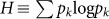for the detected symbols for both μa and μb, where pk is the probability of detecting state k. We obtain an entropy of 3.98 ± 0.03 and 3.92 ± 0.05 bits for the QKD sessions with μa and μb, respectively. Both cases are close to the theoretical maximum of 4 bits/symbol when working in a 16-dimensional Hilbert space.

The two employed MUBs are displayed here. They obey the condition that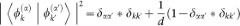, where α and α′ denote the two MUBs. k and k′ label the states in a given MUB. For sake of simplicity, we describe the MUB's states in terms of unitary transformations. The MUBs are given by: